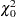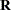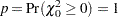### Handling the Degenerate Distribution

Likelihood ratio testing in mixed models invariably involves the chi-square distribution with zero degrees of freedom. Therandom variable is degenerate at 0, and it occurs in two important circumstances. First, it is a component of mixtures, where typically the value of the test statistic is not zero. In that case, the contribution of thecomponent of the mixture to the p-value is nil. Second, a degenerate distribution of the test statistic occurs when the null model is identical to the full model—for example, if you test a hypothesis that does not impose any (new) constraints on the parameter space. The following statements test whether thematrix in a variance component model is diagonal:

```proc glimmix;
class a b;
model y = a;
random b a*b;
covtest diagR;
run;
```

Because no R-side covariance structure is specified (all random effects are G-side effects), thematrix is diagonal in the full model and the COVTEST statement does not impose any further restriction on the parameter space. The likelihood ratio test statistic is zero. The GLIMMIX procedure computes the p-value as the probability to observe a value at least as large as the test statistic under the null hypothesis. Hence,